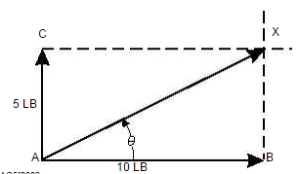# Composition of Forces

If two or more forces are acting simultaneously at apoint, the same effect can be produced by a single forceof  the  proper  size  and  direction.  This  single  force,which is equivalent to the action of two or more forces,is   called   the   resultant.   Putting   component   forcestogether to find the resultant force is called compositionof  forces.  (See  fig.  2-2.)  The  vectors  representing  theforces  must  be  added  to  find  the  resultant.  Because  avector  represents  both  magnitude  and  direction,  themethod  for  adding  vectors  differs  from  the  procedureused   for   scalar   quantities   (quantities   having   onlymagnitude and no direction). To find the resultant force when a force of 5 pounds and a force of 10 pounds are applied at a right angle to point A, refer to figure2.The   resultant   force   may   be   found   as   follows:Represent  the  given  forces  by  vectors  AB  and  ACdrawn  to  a  suitable  scale.  At  points  B  and  C  drawdashed lines perpendicular to AB and AC, respectively.From point A, draw a line to the point of intersection X,of the dashed lines. Vector AX represents the resultantofthetwoforces.Thus,whentwomutuallyperpendicular    forces    act    on    a    point,    the    vectorrepresenting  the  resultant  force  is  the  diagonal  of  arectangle. The length of AX, if measured on the samescale as that for the two original forces, is the resultantforce;  in  this  case  approximately  11.2  pounds.  Theangle  gives  the  direction  of  the  resultant  force  withrespect to the horizontal.Mathematically,theresultantforceofperpendicular   forces   can   be   found   by   using   thePythagorean theorem which deals with the solution ofright triangles. The formula is C2 = a2 + b2. This statesthat  the  hypotenuse,  side  “C”  (our  unknown  resultantforce) squared is equal to the sum of side “a” (one of ourknown  forces)  squared  and  side  “b”  (another  of  ourknown forces) squared.

### Feedback is important to us.#### Satya Prakash

Mr. Satya Prakash is a research scholar.

error: Content is protected !!